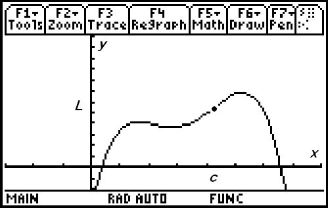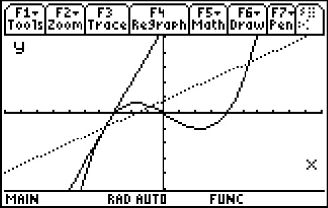# Activities

••• ##### Subject Area

• Math: Calculus: Applications of the Derivative
• Math: Calculus: Derivatives
• Math: Calculus: Applications of Integral, Antiderivatives and Definite Integrals
• Math: Calculus: Limits of Functions

• ##### Author9-12

20 Minutes

• ##### Device
• TI-89 / TI-89 Titanium

TI Connect™

• ##### Accessories

TI Connectivity Cable

## What is Calculus?#### Activity Overview

Students are introduced to the subject of calculus. This is ideal for students at the beginning of a calculus course or at the end of a precalculus course to give students an idea of the topics to come.

#### Key Steps

•Students are introduced to the subject of calculus. This is ideal for students at the beginning of a calculus course or at the end of a precalculus course to give students an idea of the topics to come.

Students will be introduced to calculus concepts that they will encounter throughout their calculus course through graphical explorations.

•